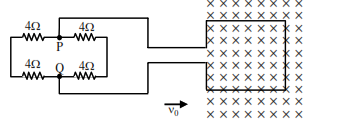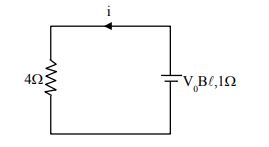# A square loop of side 20cm and resistance

Question:

A square loop of side $20 \mathrm{~cm}$ and resistance $1 \Omega$ is moved towards right with a constant speed $v_{0}$. The right arm of the loop is in a uniform magnetic field of $5 \mathrm{~T}$. The field is perpendicular to the plane of the loop and is going into it. The loop is connected to a network of resistors each of value $4 \Omega$. What should be the value of $v_{0}$ so that a steady current of $2 \mathrm{~mA}$ flows in the loop ?1. $1 \mathrm{~m} / \mathrm{s}$

2. $1 \mathrm{~cm} / \mathrm{s}$

3. $10^{2} \mathrm{~m} / \mathrm{s}$

4. $10^{-2} \mathrm{~cm} / \mathrm{s}$

Correct Option: , 2

Solution:

Equivalent circuit$\mathrm{i}=\frac{\mathrm{V}_{0} \mathrm{~B} \ell}{4+1} \Rightarrow \mathrm{V}_{0}=\frac{5(2 \mathrm{~mA})}{5 \times .2}=10^{-2} \mathrm{~m} / \mathrm{s}=1 \mathrm{~cm} / \mathrm{s}$

Option (2)